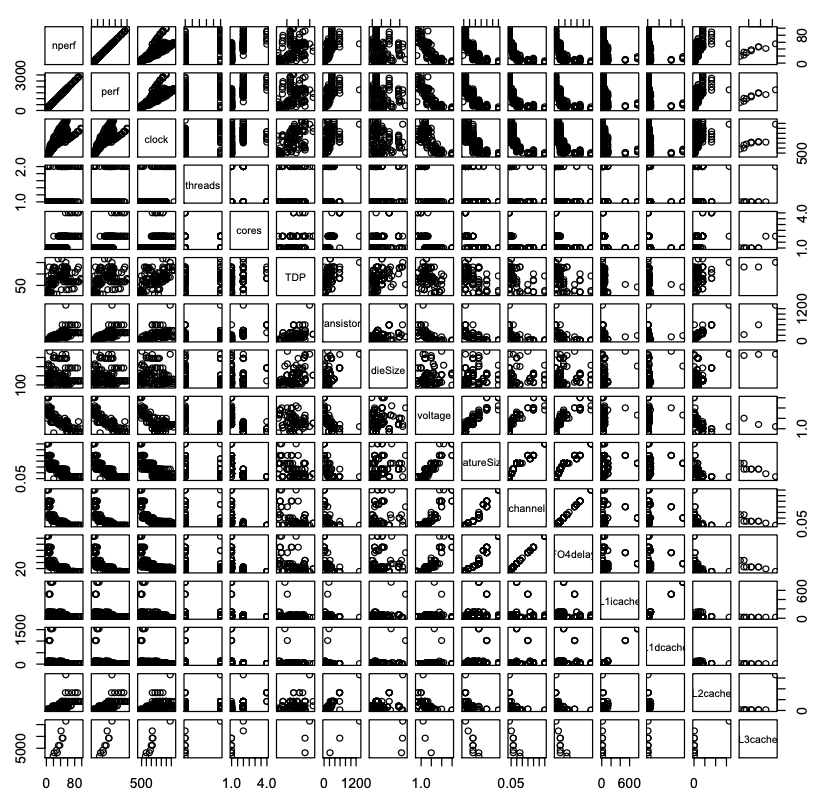# 4.1: Visualizing the Relationships in the Data

Before beginning model development, it is useful to get a visual sense of the relationships within the data. We can do this easily with the following function call:

> pairs(int00.dat, gap=0.5)

The pairs() function produces the plot shown in Figure 4.1. This plot provides a pairwise comparison of all the data in the int00.dat data frame. The gap parameter in the function call controls the spacing between the individual plots. Set it to zero to eliminate any space between plots.

As an example of how to read this plot, locate the box near the upper left corner labeled perf. This is the value of the performance measured for the int00.dat data set. The box immediately to the right of this one is a scatterFigure 4.1: All of the pairwise comparisons for the Int2000 data frame.

plot, with perf data on the vertical axis and clock data on the horizontal axis. This is the same information we previously plotted in Figure 3.1. By scanning through these plots, we can see any obviously significant relationships between the variables. For example, we quickly observe that there is a somewhat proportional relationship between perf and clock. Scanning down the perf column, we also see that there might be a weakly inverse relationship between perf and featureSize.

Notice that there is a perfect linear correlation between perf and nperf. This relationship occurs because nperf is a simple rescaling of perf. The reported benchmark performance values in the database that is, the perf values use different scales for different benchmarks. To directly compare the values that our models will predict, it is useful to rescale perf to the range [0,100]. Do this quite easily, using this R code:

max_perf = max(perf)
min_perf = min(perf)
range = max_perf min_perf
nperf = 100 * (perf min_perf) / range

Note that this rescaling has no effect on the models we will develop, because it is a linear transformation of perf. For convenience and consistency, we use nperf in the remainder of this tutorial.